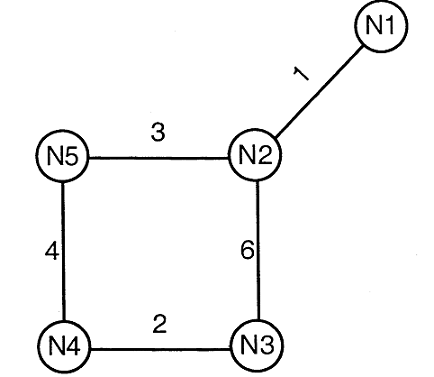GATE | GATE CS 2011 | Question 52

• Last Updated : 28 Jun, 2021

Consider a network with five nodes, N1 to N5, as shown below.The network uses a Distance Vector Routing protocol. Once the routes have stabilized, the distance vectors at different nodes are as following.

N1: (0, 1, 7, 8, 4)
N2: (1, 0, 6, 7, 3)
N3: (7, 6, 0, 2, 6)
N4: (8, 7, 2, 0, 4)
N5: (4, 3, 6, 4, 0)

Each distance vector is the distance of the best known path at the instance to nodes, N1 to N5, where the distance to itself is 0. Also, all links are symmetric and the cost is identical in both directions. In each round, all nodes exchange their distance vectors with their respective neighbors. Then all nodes update their distance vectors. In between two rounds, any change in cost of a link will cause the two incident nodes to change only that entry in their distance vectors. 52. The cost of link N2-N3 reduces to 2(in both directions). After the next round of updates, what will be the new distance vector at node, N3.
(A) (3, 2, 0, 2, 5)
(B) (3, 2, 0, 2, 6)
(C) (7, 2, 0, 2, 5)
(D) (7, 2, 0, 2, 6)